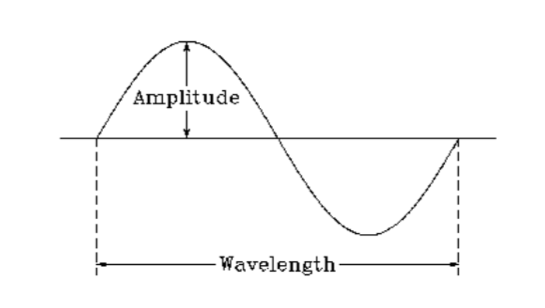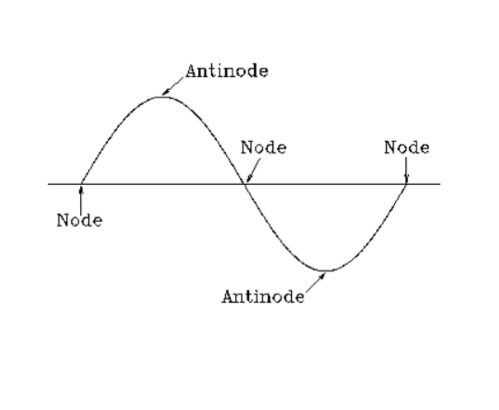# Measure the Speed of Light Using Microwaves and Chocolate

Measure the Speed of Light Using a Microwave and Chocolate

For this activity, students will be measuring the speed of light using some common household items; a microwave and chocolate. This fun and relatively simple method of measuring one of the most important numbers in scientific history demonstrates the relationship between the speed of light, frequency and wavelength both conceptually and in its mathematical form of c = fλ. What this equation tells us is that the speed of light is equal to the wavelength (λ) multiplied by the frequency (f) of an electromagnetic wave (microwaves and visible light are both examples of electromagnetic waves).

If c is what we wish to determine, we will have to know the other two variables: f and λ. The frequency f can be found on either the back of the microwave or in the microwave user manual; it will be in the units of Hertz (Hz, or MHz) which are the number of cycles per second i.e. the amount of times one complete wavelength of a wave travels past a certain point within one second.

Our experiment, therefore, is to determine our single unknown variable: the wavelength λ. Before we jump in, let’s explain how we’re going to use the chocolate to determine this value…

When a microwave is powered on, it emits microwaves which bounce back and forth between the walls of the oven. The size of the oven is chosen so that the peaks and troughs of the reflected waves line up with the incoming waves and form a “standing wave”. These standing waves have high and low points of heating (peaks and troughs) which correspond to the nodes and antinodes of the standing wave. At the antinodes, the amplitude of the wave will be at its highest and will melt the chocolate much quicker and, inversely, the nodes are where the waves cancel each other out so there is little energy to heat the chocolate and it will melt much slower.Resources Needed:

• Microwave oven
• Chocolate bar
• Plate (can be paper)
• Ruler
Duration:
60 mins

Age Suitability:
11 to 14
14 to 16
16 to 18

Teacher Files:Lesson Plan